tensorflow中相同的代码，运行多次每次的结果都不相同？ 40C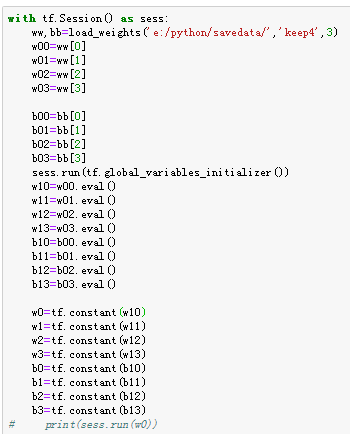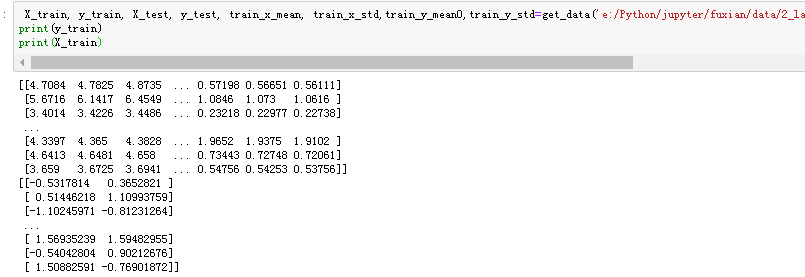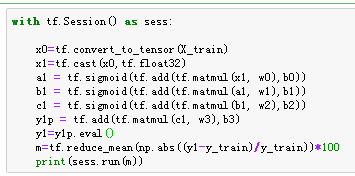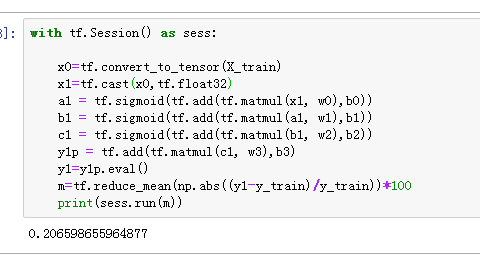# In:

import tensorflow as tf
import matplotlib.pyplot as plt

# In:

import Ipynb_importer

# In:

from core3 import *

# In:

with tf.Session() as sess:
w00=ww
w01=ww
w02=ww
w03=ww

``````b00=bb
b01=bb
b02=bb
b03=bb
sess.run(tf.global_variables_initializer())
w10=w00.eval()
w11=w01.eval()
w12=w02.eval()
w13=w03.eval()
b10=b00.eval()
b11=b01.eval()
b12=b02.eval()
b13=b03.eval()

w0=tf.constant(w10)
w1=tf.constant(w11)
w2=tf.constant(w12)
w3=tf.constant(w13)
b0=tf.constant(b10)
b1=tf.constant(b11)
b2=tf.constant(b12)
b3=tf.constant(b13)
``````

# In:

X_train, y_train, X_test, y_test, train_x_mean, train_x_std,train_y_mean0,train_y_std=get_data('e:/Python/jupyter/fuxian/data/2_layer_tio2',percentTest=.2,random_state=42)
print(y_train)
print(X_train)

# In[ ]:

with tf.Session() as sess:

``````x0=tf.convert_to_tensor(X_train)
x1=tf.cast(x0,tf.float32)
y1=y1p.eval()
m=tf.reduce_mean(np.abs((y1-y_train)/y_train))*100
print(sess.run(m))
``````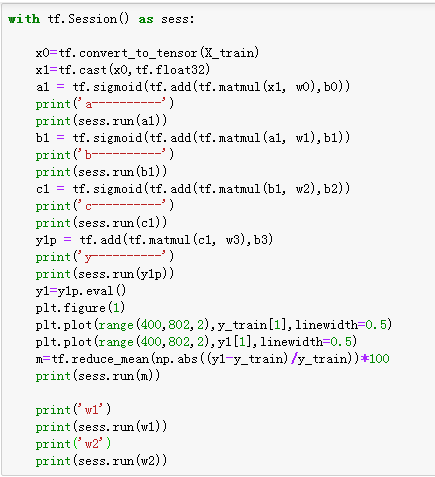2个回答

global_variables_initializer() 这个会将权重设置成随机值，你后面又没有再训练，所以结果就是随机的了。lilililizhan 权值的值我打开文档进行了对比，并没有变成随机数lilililizhan 我后面通过print(sess.run(w0))的操作，权值并没有发生变化LovePatricia 问题很简单，b1 = tf.sigmoid(tf.add(tf.matmul(a1, w1),b1)) 这一句对b1进行了重新赋值，第二次计算b1的时候值变了。把这里左边的b1换一个名字即可，同时替换后文中对应的变量lilililizhan 除了这三段代码，就是一些import的代码了